How many pumpkins were in the patch? Find out in this quiz.

# Algebra - Percentage Operations (Advancing)

This Math quiz is called 'Algebra - Percentage Operations (Advancing)' and it has been written by teachers to help you if you are studying the subject at middle school. Playing educational quizzes is a fabulous way to learn if you are in the 6th, 7th or 8th grade - aged 11 to 14.

It costs only \$12.50 per month to play this quiz and over 3,500 others that help you with your school work. You can subscribe on the page at Join Us

Some algebraic problems will ask you to find an unknown percentage increase or an unknown percentage decrease between two known factors. An unknown factor is a variable. When asked to find a percentage increase or decrease, using the following formula will help you to find the solution to your problem.

The % increase or decrease = the difference between the known factors divided by the base factor. Now let’s work this out in a practice problem below.

Go straight to Quiz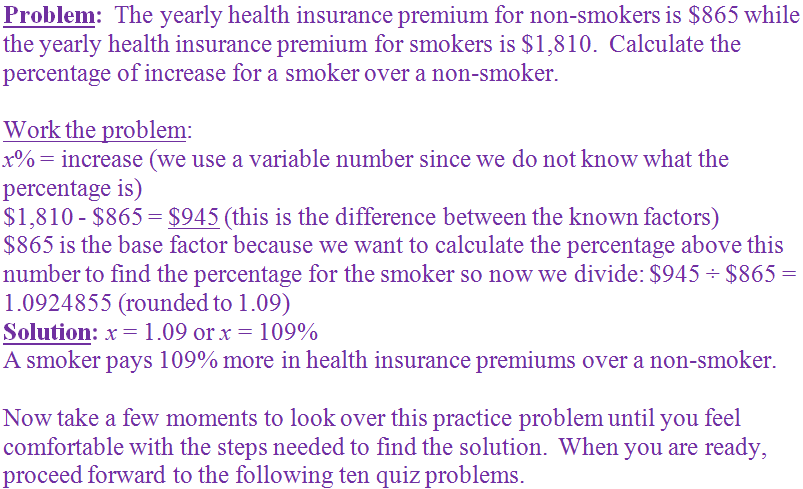1.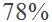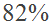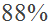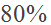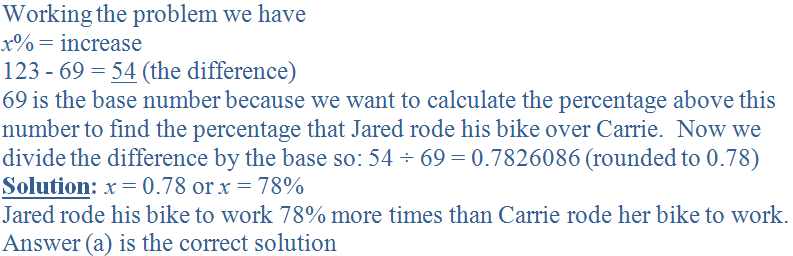2.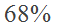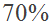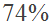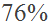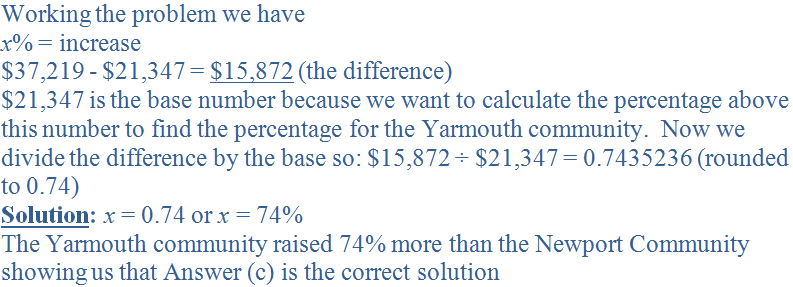3.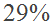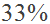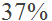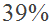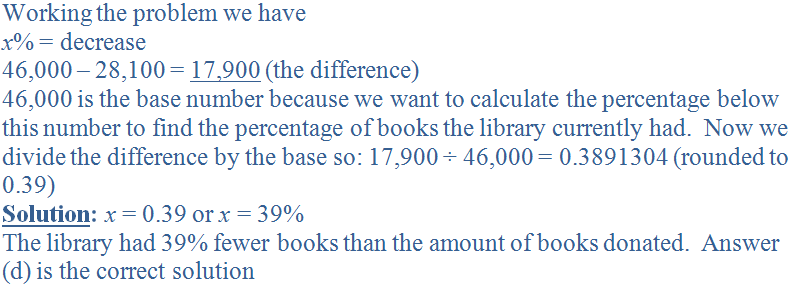4.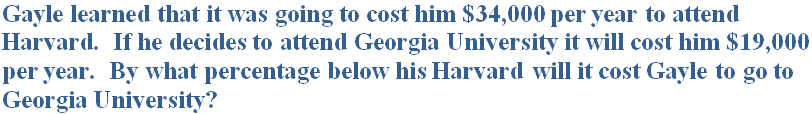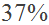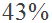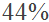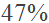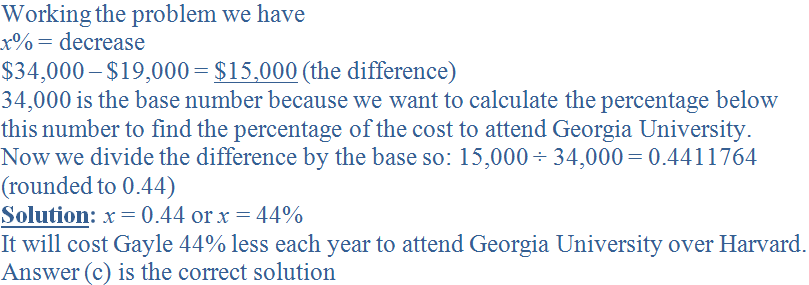5.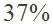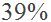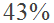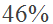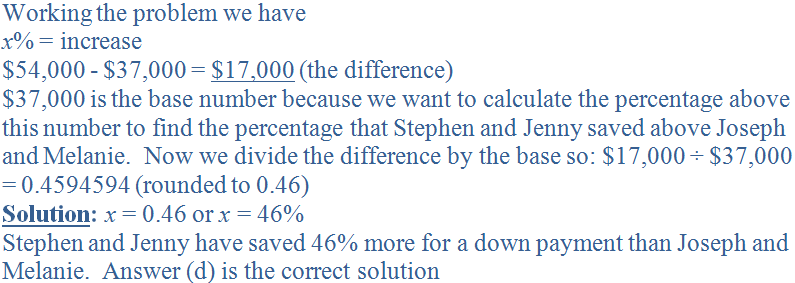6.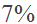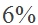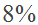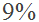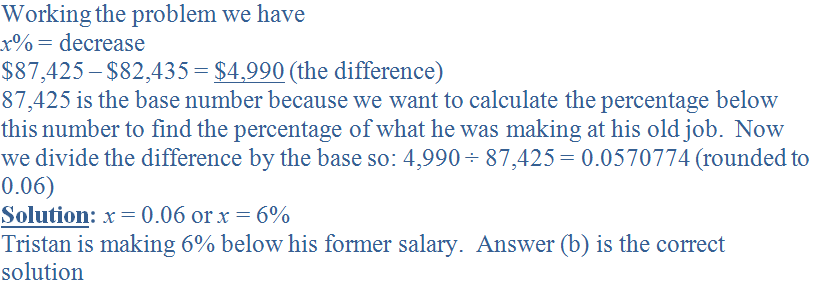7.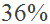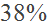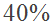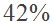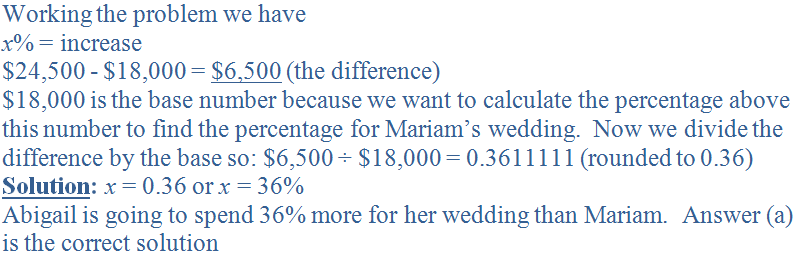8.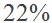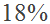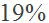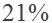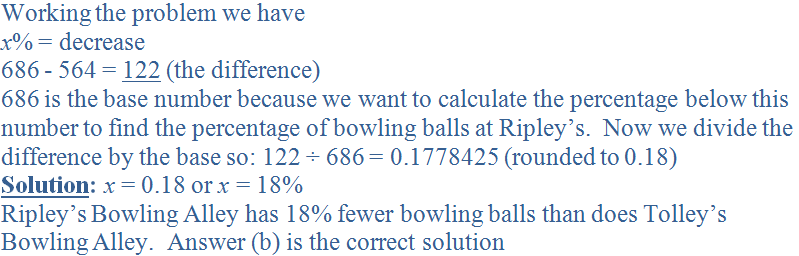9.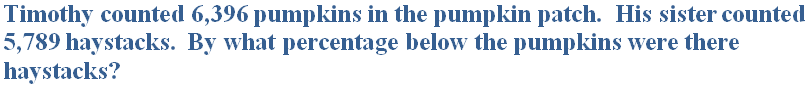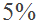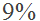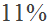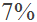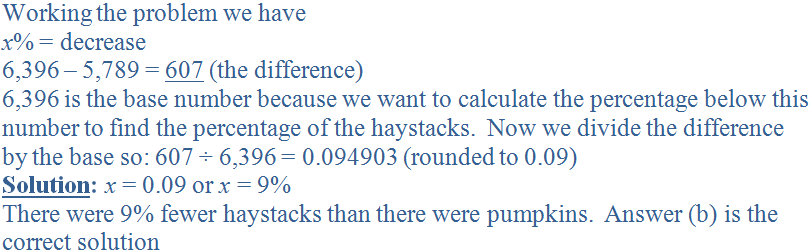10.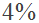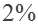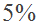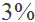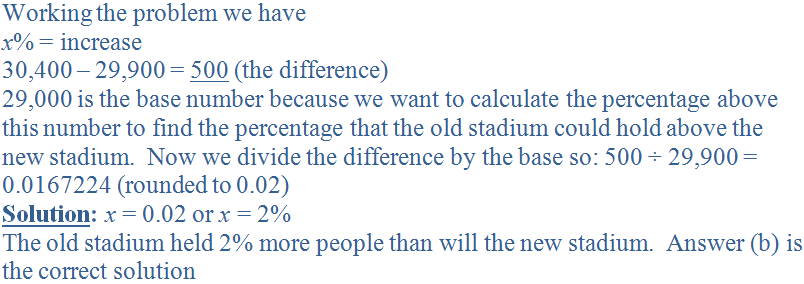Author:  Christine G. Broome